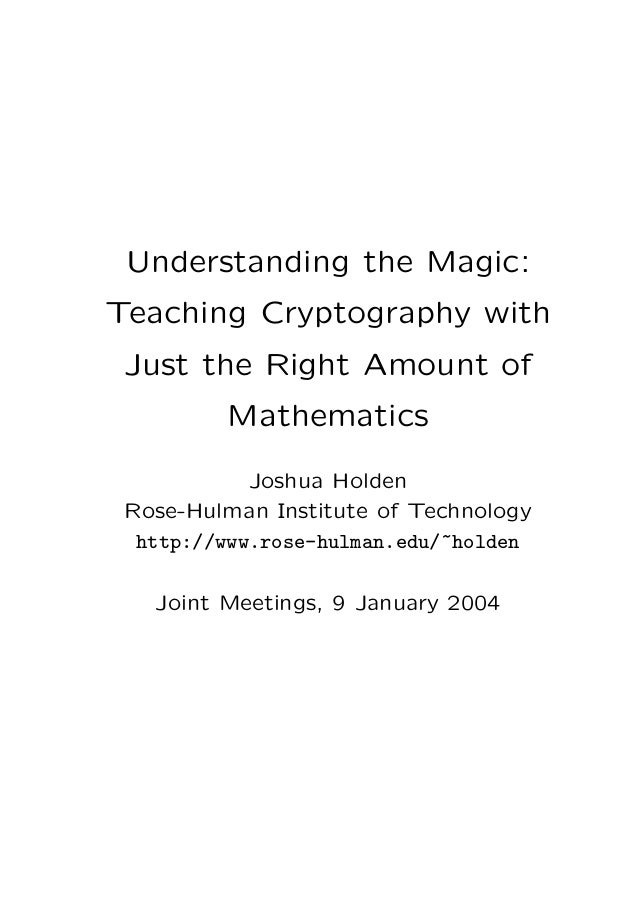Successfully reported this slideshow.
We use your LinkedIn profile and activity data to personalize ads and to show you more relevant ads. You can change your ad preferences anytime.Upcoming SlideShare
Loading in …5
×

# Understanding the Magic: Teaching Cryptography with Just the Right Amount of Mathematics

2,403 views

Published on

Cryptography is a field which has recently attracted a great deal of attention from both students and teachers of mathematics and of computer science. Students of computer science see cryptography as something which is not only "cool" but may be necessary for them to know in their future careers. Many of them do not realize, however, just how much mathematics they need to know in order to understand the algorithms which lie at the heart of modern cryptography.

Published in: Technology, Education
• Full Name
Comment goes here.

Are you sure you want to Yes No
Your message goes here• Be the first to comment

• Be the first to like this

### Understanding the Magic: Teaching Cryptography with Just the Right Amount of Mathematics

1. 1. Understanding the Magic: Teaching Cryptography with Just the Right Amount of Mathematics Joshua Holden Rose-Hulman Institute of Technology http://www.rose-hulman.edu/~holden Joint Meetings, 9 January 2004
2. 2. What am I going to show you? • Pohlig-Hellman exponentiation cipher • Fermat’s Little Theorem • Necessary ideas for RSA • One way to mix mathematics and cryptog- raphy • A neat “magic trick”? 1
3. 3. What comes before? • Shift ciphers • Modular arithmetic • Multiplicative ciphers • Euclidean algorithm and multiplicative in- verses • Block ciphers (e.g. Hill cipher) • Private-key vs. public-key ciphers 2
4. 4. Pohlig-Hellman exponentiation cipher (1978) • Private key block cipher • m letters → 2m-digit number • Example: m = 2, blocks are 4 digit num- bers 2 ca → 0200 2 ts → 1918 2 an → 0013 2 dd → 0303 2 og → 1406 2 sx → 1803 3
5. 5. Encryption • Pick a prime number larger than the largest possible block • e.g. p > 2525, say p = 3001 • Pick a key, e • Shift ciphers: add key. Multiplicative ciphers: multiply by key. Exponential cipher: C ≡ Pe mod p. (C is ciphertext block; P is plaintext block) 4
6. 6. • Let e = 7, then 2 (0200)7 ≡ 1640 mod 3001 2 (1918)7 ≡ 0213 mod 3001 2 (0013)7 ≡ 0608 mod 3001 2 (0303)7 ≡ 1140 mod 3001 2 (1406)7 ≡ 2918 mod 3001 2 (1823)7 ≡ 0094 mod 3001 5
7. 7. Decryption • Now we have ciphertext. Recipient needs to recover plaintext P. • Need to be able to take e-th roots! • C1/e mod p doesn’t make sense. • Need an inverse of some sort. What sort? • Need a number d such that Cd ≡ P mod p ⇔ (Pe)d ≡ P mod p ⇔ Ped ≡ P mod p ⇔ PedP−1 ≡ 1 mod p ⇔ Ped−1 ≡ 1 mod p • So it’s very important to know what num- bers x have Px ≡ 1 mod p 6
8. 8. Theorem (Fermat’s Little Theorem, 1640) If p is a prime number and a is a positive integer, p a, then ap−1 ≡ 1 mod p. “Proof by Example” 36 ≡ 1 mod 7 Start with 1,2,3,4,5,6. Multiply each by 3 mod 7: 3,6,2,5,1,4. Second row is a rearrangement of the ﬁrst! So 1 · 2 · 3 · 4 · 5 · 6 ≡ 3 · 6 · 2 · 5 · 1 · 4 mod 7 ≡ (3 · 1)(3 · 2)(3 · 3)(3·)(3 · 5)(3 · 6) mod 7 ≡ 36 · 1 · 2 · 3 · 4 · 5 · 6 mod 7. All of k = 1, 2, 3, 4, 5, 6 have gcd(k, 7) = 1, so can multiply by their inverses to cancel. Thus 1 ≡ 36 mod 7 Real Proof (if desired) (pretty much the same!) 7
9. 9. Now what do we need to decrypt? • Need Ped−1 ≡ 1 mod p • This works if ed−1 = k(p−1) because then Ped−1 ≡ Pk(p−1) mod p ≡ (P(p−1))k mod p ≡ 1k mod p ≡ 1 mod p • ed − 1 = k(p − 1) means ed ≡ 1 mod p − 1 ⇔ d ≡ e mod p − 1 (not mod p!) • So for decryption we ﬁgure out d ≡ e mod p − 1 using gcd(e, p − 1), which had better = 1 and let P ≡ Cd ≡ Ce mod p 8
10. 10. • Luckily, if p = 3001, e = 7, then gcd(7, 3000) = 1 so we can decrypt • d ≡ e ≡ 2143 mod p − 1 = 3000 (using the Euclidean algorithm) 2 (1640)2143 ≡ 0200 mod 3001 → ca 2 (0213)2143 ≡ 1918 mod 3001 → ts 2 (0608)2143 ≡ 0013 mod 3001 → an 2 (1140)2143 ≡ 0303 mod 3001 → dd 2 (2918)2143 ≡ 1406 mod 3001 → og 2 (0094)2143 ≡ 1823 mod 3001 → sx • Magic! But now you know the trick. 9
11. 11. What comes next? • Security: the discrete log problem • Exponentiation with composite moduli • Where Fermat’s Little Theorem breaks • The Euler phi function • Euler’s Theorem • RSA • Fast exponentiation • Fast primality testing • . . . 10